Compton Scattering

Yet another experiment which illustrated the photon nature of light was performed in 1923 by Compton, and is called Compton scattering. In this experiment light is scattered off of an electron in a material, as in Fig. 27.5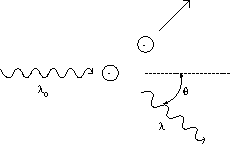It is found that the scattered light has a wavelength different than that of the incident light, an effect that again cannot be explained using a wave picture of light, where the wavelength does not change. Compton analyzed this problem by considering light as a photon with energy given by Planck's hypothesis, Eq.(27.5), as well as a momentum given by

 p =, (8)

which was motivated in part by Einstein's theory of Special Relativity. Using the energy and momentum conservation for such a collision, he found the following relation between the incident and scattered photon: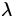=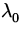+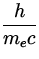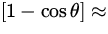+ 0.0024nm , (9)

where me = 9.11 x 10- 31 kg is the mass of the electron. Such a small difference in wavelengths between the incident and scattered light is very hard to detect using visible light, with wavelengths of the order of 500 nm. However, it is a comparatively large effect for X-rays, which have wavelengths of the order of 0.1 nm, and so X-rays were used to demonstrate that such scattering does indeed take place.Next: Wave-Particle Duality Up: Quantum Physics Previous: The Photoelectric Effect